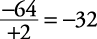## Signed Numbers (Positive Numbers and Negative Numbers)

The term signed numbers refers to positive and negative numbers. If no sign is shown, the number automatically is considered positive.

#### Number lines

On a number line, numbers to the right of 0 are positive. Numbers to the left of 0 are negative, as shown in Figure 1.

Figure 1. A number line using integers.Given any two numbers on a number line, the one on the right is always larger, regardless of its sign (positive or negative). Note that fractions may also be placed on a number line as shown in Figure 2.

Figure 2. A number line using fractions.When adding two numbers with the same sign (either both positive or both negative), add the absolute values (the number without a sign attached) and keep the same sign. Addition problems can be presented in either a vertical form (up and down) or in a horizontal form (across).

##### Example 1

1.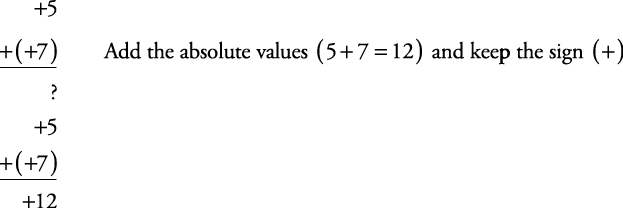2.When adding two numbers with different signs (one positive and one negative), subtract the absolute values and keep the sign of the one with the larger absolute value.

##### Example 2

1.2.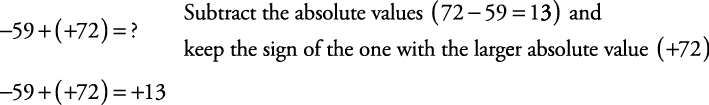##### Example 3

1.2.3.4.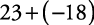1. 15

2. 3

3. 3

4. 5

#### Subtraction of signed numbers

To subtract positive and/or negative numbers, just change the sign of the number being subtracted and then add.

##### Example 4

Subtract the following.

1.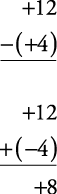2.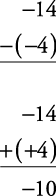3.4.Subtracting positive and/or negative numbers may also be done “horizontally.”

##### Example 5

Subtract the following.

1. +12 – (+4) = +12 + (–4) = 8

2. +16 – (–6) = +16 + (+6) = 22

3. –20 – (+3) = –20 + (–3) = –23

4. –5 – (–2) = –5 + (+2) = –3

#### Minus preceding parenthesis

If a minus precedes a parenthesis, it means everything within the parentheses is to be subtracted. Therefore, using the same rule as in subtraction of signed numbers, simply change every sign within the parentheses to its opposite and then add.

##### Example 6

Subtract the following.

1.2.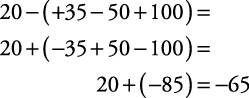#### Multiplying and dividing signed numbers

To multiply or divide signed numbers, treat them just like regular numbers but remember this rule: An odd number of negative signs will produce a negative answer. An even number of negative signs will produce a positive answer.

##### Example 7

Multiply or divide the following.

1. (–3)(+8)(–5)(–1)(–2) = +240

2. (–3)(+8)(–1)(–2) = –48

3.4.